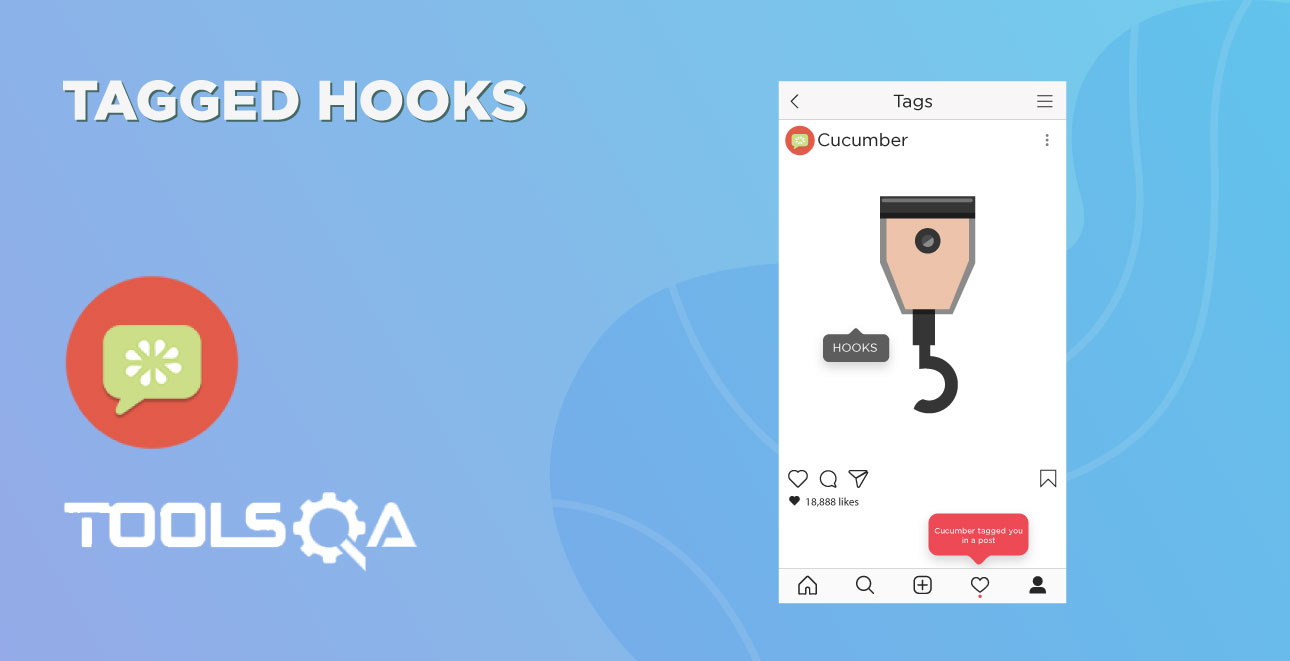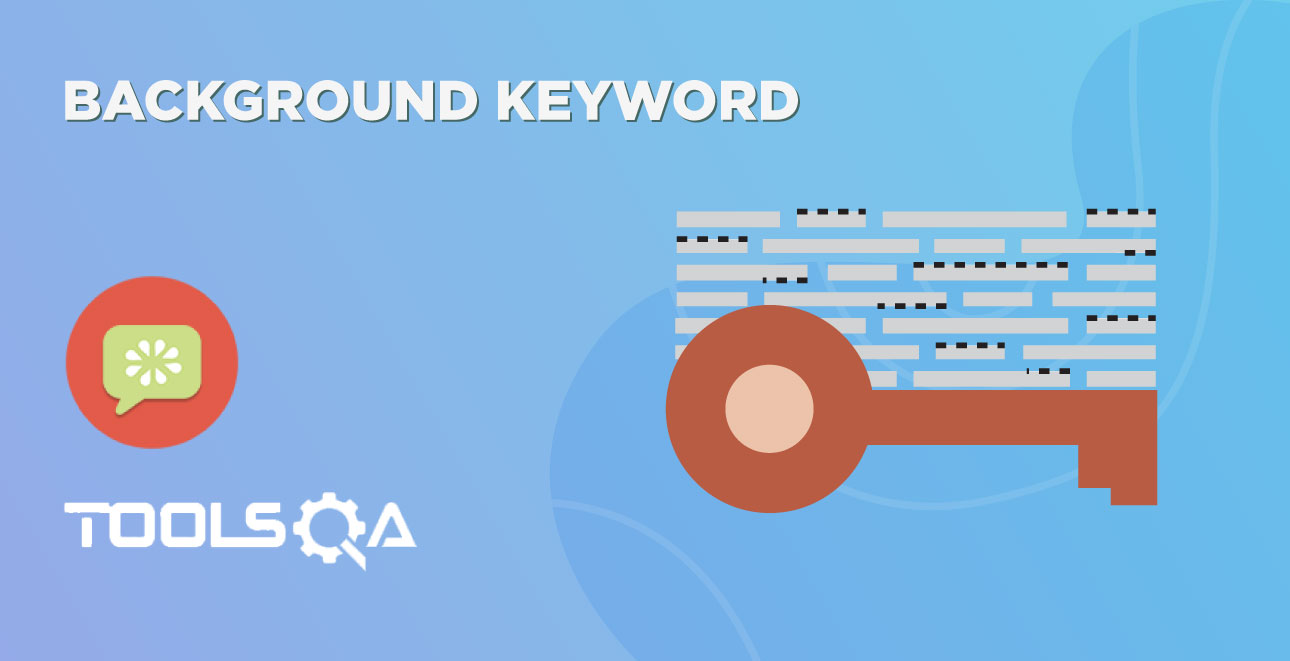In this chapter, we will learn about Execution Order of Hooks. If you ever have worked with TestNG, you must know that it performs the execution in a certain order. The same way Cucumber also executes the hooks in a certain order. But there are ways to change the order of the executing according to the need of the test or the framework.

Before moving to this chapter, you must know about the Cucumber Tags, Cucumber Hooks and Tagged Hooks in Cucumber.

## Execution Order of Hooks

Order hooks to run in a particular sequence is easy to do. As we already know the way to specify hooks in cucumber-like putting an annotation just above the scenario. Ordering also works the same way but the only difference is that it required an extra parameter. This extra parameter decides the order of execution of the certain hook.

For example @Before, and if you want to specify the order it will become @Before(value = 1).

The same goes with any Tags or Hooks available in Cucumber including Tagged Hooks as well.

### Exercise on Order Hooks

Let's take a different approach this time and do an exercise with the multiple hooks without any ordering value. Later we will bring order value and see the difference in output.

Feature File

``````Feature: Test Order Hooks

Scenario: First scenario to test Order Hooks functionality
Given this is the first step
When this is the second step
Then this is the third step

Scenario: Second scenario to test Order Hooks functionality
Given this is the first step
When this is the second step
Then this is the third step

``````

This is the same plain feature file that we used in previous chapters on Tags, Hooks, and Tagged Hooks.

Step Definitions

``````package stepDefinition;
import cucumber.api.java.en.Given;
import cucumber.api.java.en.Then;
import cucumber.api.java.en.When;

public class Hooks_Steps {
@Given("^this is the first step\$")
public void This_Is_The_First_Step(){
System.out.println("This is the first step");
}
@When("^this is the second step\$")
public void This_Is_The_Second_Step(){
System.out.println("This is the second step");
}
@Then("^this is the third step\$")
public void This_Is_The_Third_Step(){
System.out.println("This is the third step");
}
}
``````

Again, steps definitions are also same as previous chapters.

Hooks

``````package utilities;
import cucumber.api.java.After;
import cucumber.api.java.Before;

public class Hooks {

@Before
public void beforeScenario(){
System.out.println("This will run before the every Scenario");
}
@Before
public void beforeScenarioStart(){
System.out.println("-----------------Start of Scenario-----------------");
}
@After
public void afterScenarioFinish(){
System.out.println("-----------------End of Scenario-----------------");
}
@After
public void afterScenario(){
System.out.println("This will run after the every Scenario");
}

}
``````

Above we mentioned two before and two after hooks. Execute the feature file as a whole and see the output below.

Output

``````Feature: Test Order Hooks
-----------------Start of Scenario-----------------
This will run before the every Scenario
This is the first step
This is the second step
This is the third step
-----------------End of Scenario-----------------
This will run after the every Scenario
-----------------Start of Scenario-----------------
This will run before the every Scenario
This is the first step
This is the second step
This is the third step
-----------------End of Scenario-----------------
This will run after the every Scenario
``````

I would say that I want -----End of Scenario------ to be printed after the This will run after the every Scenario.

### How to set the Order or Priority of Cucumber Hooks?

The very important thing to note here is:

• @Before(order = int) : This runs in increment order, means value 0 would run first and 1 would be after 0.
• @After(order = int) : This runs in decrements order, means apposite of @Before. Value 1 would run first and 0 would be after 1.

So, as per the logic above the Hooks file will look like below.

Hooks

``````package utilities;

import cucumber.api.java.After;
import cucumber.api.java.Before;

public class Hooks {

@Before(order=1)
public void beforeScenario(){
System.out.println("This will run before the every Scenario");
}
@Before(order=0)
public void beforeScenarioStart(){
System.out.println("-----------------Start of Scenario-----------------");
}

@After(order=0)
public void afterScenarioFinish(){
System.out.println("-----------------End of Scenario-----------------");
}
@After(order=1)
public void afterScenario(){
System.out.println("This will run after the every Scenario");
}

}
``````

Output

``````Feature: Test Order Hooks
-----------------Start of Scenario-----------------
This will run before the every Scenario
This is the first step
This is the second step
This is the third step
This will run after the every Scenario
-----------------End of Scenario-----------------
-----------------Start of Scenario-----------------
This will run before the every Scenario
This is the first step
This is the second step
This is the third step
This will run after the every Scenario
-----------------End of Scenario-----------------
``````

Now just play around with the Hooks + Order, also try to figure out how it behaves when you use the Ordering with Tagged Hooks.ReviewersVirender Singh